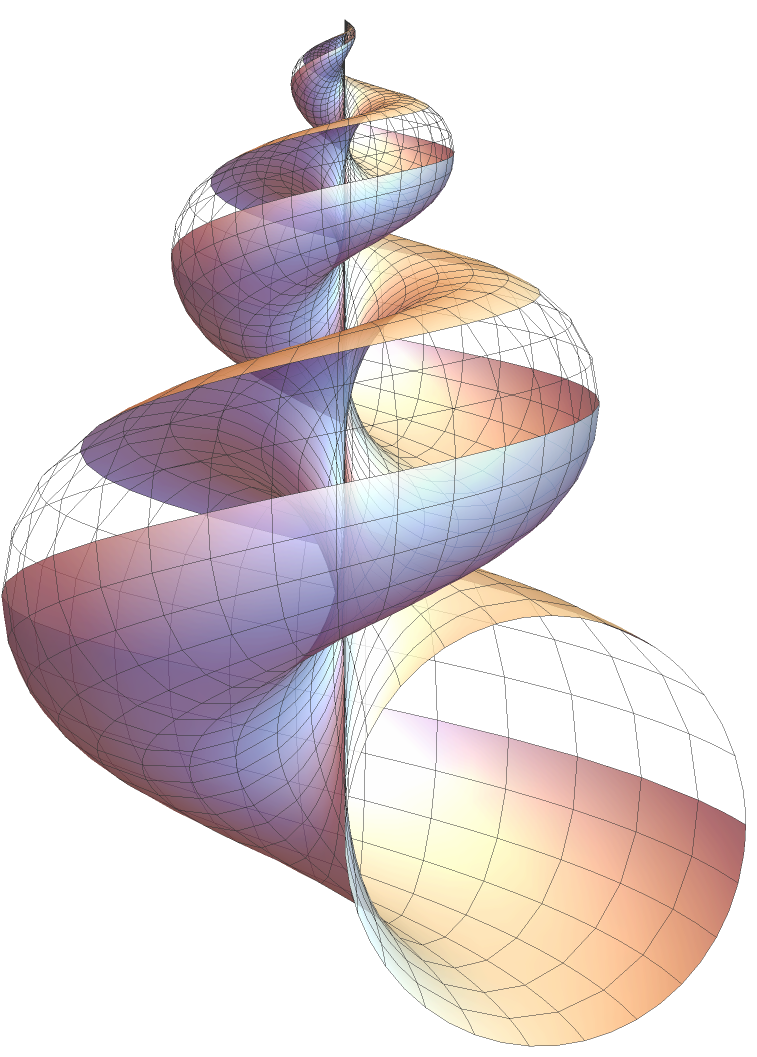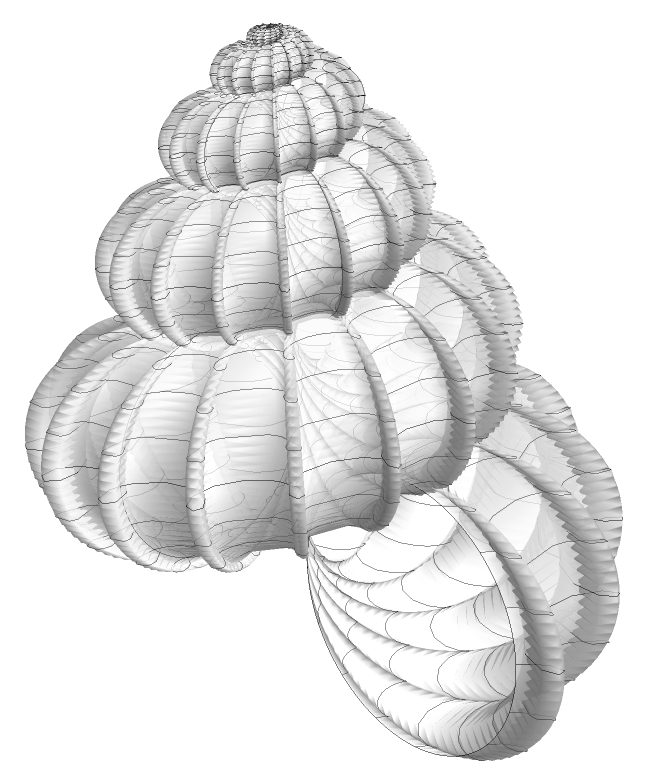# Math of Seashell Shapes

By Xah Lee. Date: . Last updated: .A mathematical model of the simplest seashell shape.

A simple seashell can be modeled using the following parametric formula:

```seashell[u_, v_] := {2*(1 - E^(u/(6*Pi)))*Cos[u]*
Cos[v/2]^2, 2*(-1 + E^(u/(6*Pi)))*Cos[v/2]^2*
Sin[u], 1 - E^(u/(3*Pi)) - Sin[v] +
E^(u/(6*Pi))*Sin[v]};

start = 0;
end = 2*Pi;
gap = 1;
shift = 1;
uMin = 0;
uMax = 6*Pi;
xViewPoint = {1.0992, 3.2001, 0.0255};

gra1 = ParametricPlot3D[Evaluate[seashell[u, v]],
{u, uMin, uMax}, {v, end - gap + shift, end + shift},
PlotPoints -> {96, 4},
Mesh -> Full,
PlotRange -> All
]

gra2 = ParametricPlot3D[Evaluate[seashell[u, v]],
{u, uMin, uMax}, {v, start + shift, end - gap + shift},
PlotPoints -> {96, 20},
Mesh -> Full,
PlotStyle -> Directive[White, Opacity[.8], Specularity[White, 20]],
PlotRange -> All
]

Graphics3D[{ gra1[] , gra2[]},
{PlotRange -> All, Boxed -> False,
ViewPoint -> xViewPoint}]```

### wrinkled periwinklewrinkled periwinkle 2022-01-21 Formula from Mike Williams mike@econym.demon.co.uk, 2005 Jan.
```cR=1; "* radius of tube *";
cN=4.6;  "* number of turns *";
cH=2.5; "* height *";
cF=80; "* wave frequency *";
cA=0.2; "* wave amplitude *";
cP=1.9; "* power *";

cW = ((#/(2*Pi)*cR) &);

seashellF = Function[ {u, v}, {
cW[u]*Cos[cN*u]*(1+Cos[v]+Cos[cF*u]*cA) ,
cW[u]*Sin[cN*u]*(1+Cos[v]+Cos[cF*u]*cA) ,
cW[u]*Sin[v] + cH*(u/(2*Pi))^cP
} ];

ParametricPlot3D[
Evaluate@  seashellF[u, v],
{u, 0, 5},
{v, 0, 2 Pi},
PlotPoints -> {160, 10},
PlotRange -> All,
ColorFunction -> "TemperatureMap",
BoundaryStyle -> Directive[Black, Thin],
PlotStyle -> Directive[White, Opacity[0.7], Specularity[10, 20]],
Lighting -> "Neutral"
]```

### Wentletrapwentletrap Formula from Mike Williams mike@econym.demon.co.uk, 2005 Jan.
```Clear[cW, seashell, cN, cH, cP, cA, cF]

cN = 6.6; ("number of turns");
cH = 4.0; ("height");
cP = 2; (" power ");
cA = 0.12;(" Ridge Amplitude ");
cF = 15;(" Ridge Frequency ");

cW = Function[(#/(2 Pi))^#2 ];

seashell[u_, v_] := {cW[u , cP] (Cos[cN u] - cA Cos[cN cF u]) (1 + Cos[v]),
cW[u , cP] (Sin[cN u] + cA Sin[cN cF u]) (1 + Cos[v]),
cW[u , cP] Sin[v] + cH (u/(2 Pi))^(1 + cP)};

ParametricPlot3D[
Evaluate@ seashell[u, v] ,
{u, 0, 6},
{v, 0, 2 Pi},
PlotPoints -> {200,40},
Axes -> True,
Boxed -> True,
BoundaryStyle -> Directive[Black, Thin],
PlotStyle -> Directive[White, Opacity[0.7], Specularity[10, 20]],
PlotRange -> All,
Lighting -> "Neutral"
]```

### Analysis of Seashell Shapes

Seashells are showcasing of spirals. There are great variety of spiral shapes. Suppose we start with a circle winding around a spiral.

• The circle's shape changes periodically like a sine function, creating a corrugated shell somewhat emulate that of

• If instead of a circle we have a triangle, we can simulate that of

• If the rounding shape periodically changes shape to have spikes, then we might emulate shells that have horns such as the

or

• The periodic change might also emulate those shell having ribs such as the

### References and Sources

The Algorithmic Beauty of Sea Shells , by Hans Meinhardt, Przemyslaw Prusinkiewicz, Deborah R Fowler. Buy at amazon

Mike Willams has sent me various formulas, see Math Parametric Equation for Seashell